# Binder cumulant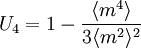$U_4 = 1- \frac{\langle m^4 \rangle }{3\langle m^2 \rangle^2 }$
where m is the order parameter. It is therefore a fourth order cumulant, related to the kurtosis. In the thermodynamic limit, where the system size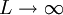$L \rightarrow \infty$,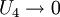$U_4 \rightarrow 0$ for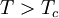$T > T_c$, and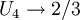$U_4 \rightarrow 2/3$ for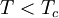$T < T_c$. Thus, the function is discontinuous in this limit. The useful fact is that curves corresponding to different system sizes (which are, of course, continuous) all intersect at approximately the same temperature, which provides a convenient estimate for the value of the critical temperature.